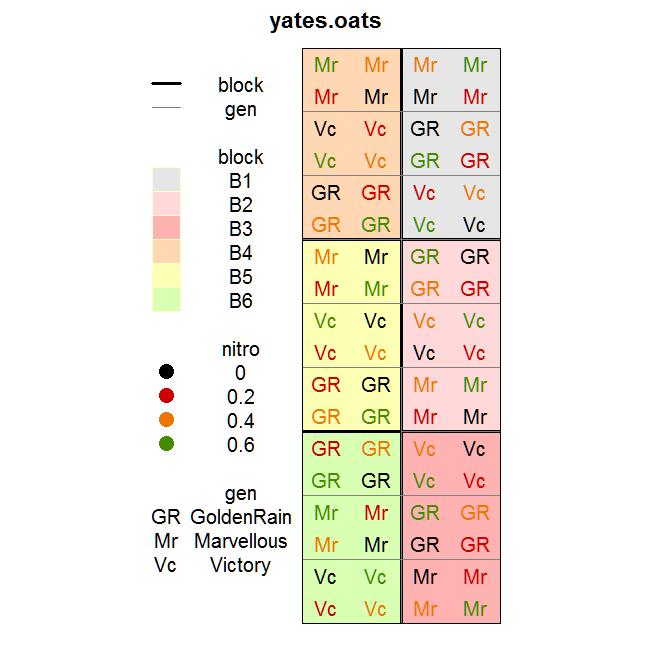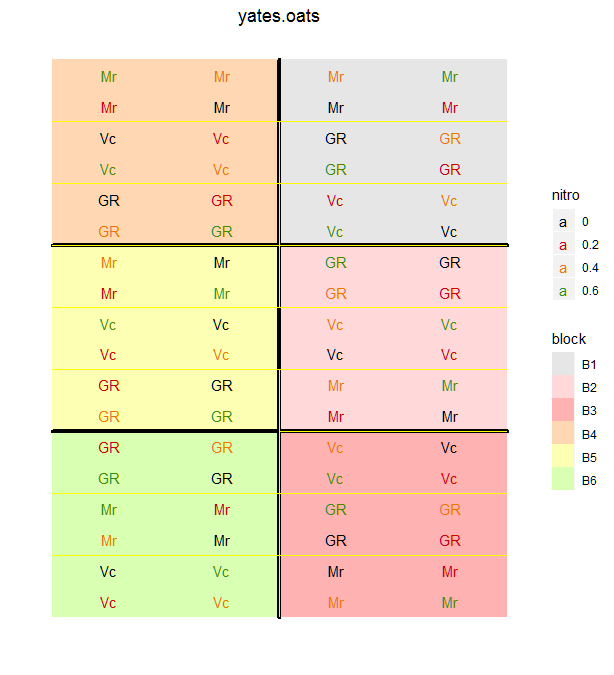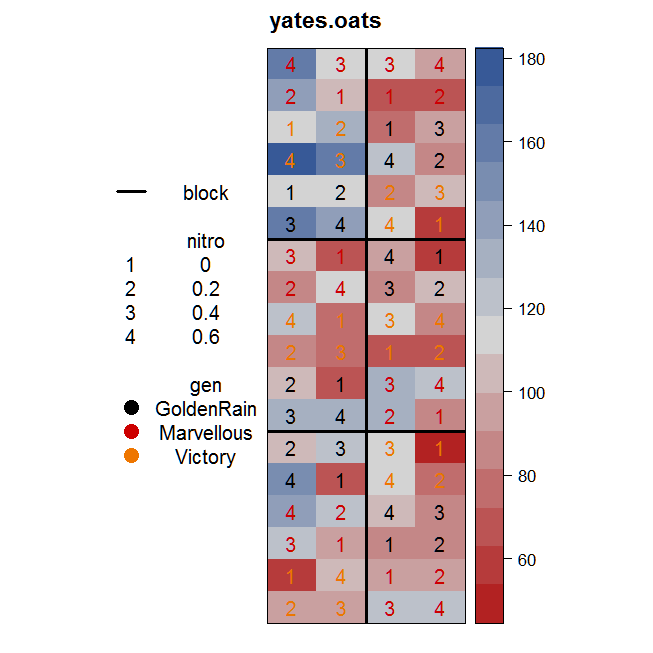Homepage: https://kwstat.github.io/desplot

Repository: https://github.com/kwstat/desplot

desplot makes it easy to plot experimental designs of field trials in agriculture.

Key features:

• Flexible options to customize appearance of graphic.

• Stable, well-tested using lattice graphics.

• Beta version using ggplot2 graphics. Note ggplot2 is about 4 times slower.

## Installation

# Install the released version from CRAN:
install.packages("desplot")

# Install the development version from GitHub:
install.packages("devtools")
devtools::install_github("kwstat/desplot")

## Example 1

This data is is from a split-plot experiment with 6 replicates. The replicates are shown by the colored regions and outlined by the thick lines. The text codes and the thin lines define the whole-plots. The nitrogen sub-plot treatments are shown by the text colors.

require(agridat)
require(desplot)
desplot(yates.oats, block ~ col+row,
col=nitro, text=gen, cex=1, aspect=511/176,
out1=block, out2=gen,
out2.gpar=list(col = "gray50", lwd = 1, lty = 1))The default graphics are based on lattice. It is also possible to create graphics based on ggplot2 by adding ‘gg=TRUE’ to the function. This functionality is in development and the legend for the ggplot version is not as polished as the lattice version.

require(agridat)
require(desplot)
desplot(yates.oats, block ~ col+row,
col=nitro, text=gen, cex=1, aspect=511/176,
out1=block, out2=gen,
out2.gpar=list(col = "gray50", lwd = 1, lty = 1), gg=TRUE)
# note, out2.gpar is ignored## Example 2

Another very useful technique is to color the cells according to a continuous response variable (such as plot yield).

require(agridat)
require(desplot)
desplot(yates.oats, yield ~ col*row,
col=gen, num=nitro, cex=1, out1=block, aspect=511/176)